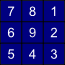# Aspect

This tool calculates slope aspect (i.e. slope orientation in degrees) for each grid cell in an input digital elevation model (DEM). The user must specify the name of the input DEM and the output aspect raster image. The Z Conversion Factor is only important when the vertical and horizontal units are not the same in the DEM. When this is the case, the algorithm will multiply each elevation in the DEM by the Z Conversion Factor. Aspect grids are best visualized using the circular_bw palette.

## Algorithm Description

The tool uses Horn's (1981) 3rd-order finite difference method to estimate slope. Given the following clock-type grid cell numbering scheme (Gallant and Wilson, 2000),the tool calculates aspect as:

aspect = 180 - arctan(fy / fx) + 90(fx / |fx|)

where

fx = (z3 - z5 + 2(z2 - z6) + z1 - z7)

and

fy = (z7 - z5 + 2(z8 - z4) + z1 - z3)

Aspect is represented as an azimuth clockwise from north, ranging from 0-360 degrees.

``` wd = pluginHost.getWorkingDirectory() inputFile = wd + "DEM.dep" outputFile = wd + "aspect.dep" zFactor = "1.0" args = [inputFile, outputFile, zFactor] pluginHost.runPlugin("Aspect", args, False) ```
``` def wd = pluginHost.getWorkingDirectory() def inputFile = wd + "DEM.dep" def outputFile = wd + "aspect.dep" def zFactor = "1.0" String[] args = [inputFile, outputFile, zFactor] pluginHost.runPlugin("Aspect", args, false) ```